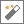After the calculation is completed the result of the math operation can be obtained using the Variable Wizard. Both fixed number or dynamic Variable Wizard values can be used to set both input numbers.

MathDetails
Number A number in decimal format, e.g., `123`, or in a scientific E notation, e.g., `1.6726219e-27` or `2.99792458e+8` numbers.
Operation Choose the mathematical operation:
• Plus
• Minus
• Multiply
• Divide
• Square root
• Modulo · returns the remainder of a division.
2nd number All the operations (except the square root) require a second argument or input value.
Decimal places Select number of decimal places for the output of the arithmetic operation. Automation Workshop supports very large and extremely precise numbers.
Variable WizardUse dynamic data input—substitute a parameter from a file, web, connected Trigger, other Actions, date and time presets, etc.

## Examples…

See some math examples with a possible output:

• Calculated 18.786 + 365.335 = 384.121
• Calculated 18.786 - 365.335 = -346.549
• Calculated 18.786 × 17.21 = 323.307
• Calculated 2 ÷ 3 = 0.67
• Calculated 2 ÷ 3 = 0.6666666666666667
• Calculated 4.5e-06 ÷ -4.53e-08 = -99.33774834437086092715
• Calculated √2 = 1.41
• Calculated √2 = 1.41421356237309504880168872421
• Calculated √2 = 1.414213562373095048801688724209698078569716913762
• Calculated 10 mod 3 = 1
• Calculated -11.11 mod 3 = -2.11
• Calculated 12345678901234567890 mod 971.113547743313 = 452.2923038239
• Calculated √-17 = nan
• Calculated 42 ÷ 0 = ∞

## Have a question?

If you have any questions, please do not hesitate to contact our support team.